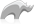##### [ staticarray->find ]

Method

[staticarray->find] returns an array of elements that match the parameter.

Accepts a single parameter of any data type. If the array contains any pair values, only the first part of the pair is compared with the parameter of the [staticarray->find] method. An optional offset parameter may be added to determine the staring point in the static array for comparison.

If no elements in the array match the parameter to the [staticarray->find] method then an empty array is returned.

Note: This method returns objects of type array.

• Syntax
``````staticarray->find('find_value')

staticarray->find('find_value', offset)``````
• Beginner

### To find an element in a staticarray of pairs:

Use the [staticarray->find] method with the value of the first element of the pairs that should be returned from the array.

The following example shows an array of pairs returned by the [staticarray->find] method, each of which has a first element of 'John Doe'.

Code

``````var( People_Array = staticarray('John Doe' = 'Person One',
'Jane Doe' = 'Person Two',
'Joe Surname' = 'Person Three',
'John Doe' = 'Person Four') )

\$People_Array->find('John Doe')``````

Result

`array((John Doe = Person One), (John Doe = Person Four))`

To find an element in a staticarray of pairs starting at a given offset position

The following example shows uses the [staticarray->find] method to return an array of pairs which begin with 'John Doe' starting from the second element of the original array.

Code

``````var( People_Array = staticarray('John Doe' = 'Person One',
'Jane Doe' = 'Person Two',
'Joe Surname' = 'Person Three',
'John Doe' = 'Person Four') )

\$People_Array->find('John Doe', 2)``````

Result

`array((John Doe = Person Four))`Please note that periodically LassoSoft will go through the notes and may incorporate information from them into the documentation. Any submission here gives LassoSoft a non-exclusive license and will be made available in various formats to the Lasso community.